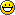^ 回到顶部
• 人生没有定律，每个人都有自己的节奏
• 本站wordpress建站教程均通过实践后发布，希望对你有帮助
• 希望你的坚持，都是因为热爱，而不是因为不甘心
• 5年wordpress建站经验，5星服务品质
• 那些不愿意让你吃亏的人，才是真正值得你深交的人，也是值得你付出时间的人

# 零起点php入门第4课-php常用运算符（2）1.取反运算符

2.四则运算符

PHP 中的算数运算符同数学非常类似，最常用的例如+（加法运算符）、-（减法运算符）、*（乘法运算符）、/（除法运算符）运算符的使用方法同数字中的四则运算符相同。

```<?php
\$x=10+15;			//加法运算
\$y=50-\$x;			//减法运算
\$z=\$y*2;			//乘法运算
\$n=\$z/5;			//除法运算
echo \$x;			//输出变量 x 的值
echo \$y;			//输出变量 y 的值
echo \$z;			//输出变量 z 的值
echo \$n;			//输出变量 n 的值
?>```

3.取模运算符

```<?php
\$x=10%5;			//进行求余运算
\$y=10%3;			//进行求余运算
\$z=10%6;			//进行求余运算
echo \$x;			//输出变量 x 的值
echo \$y;			//输出变量 y 的值
echo \$z;			//输出变量 z 的值
?>```

4.复合赋值运算符

```<?php
\$x=10;			//初始化变量 x
echo \$x;		//输出变量 x 的值
\$x=\$x+5;		//将变量 x 原来的值加 5 后赋值给自身
echo \$x;		//输出变量 x 的值
\$x=\$x*5;		//将变量 x 原来的值乘以 5 后赋值给自身
echo \$x;		//输出变量 x 的值
\$x=\$x/5;		//将变量 x 原来的值除以 5 后赋值给自身
echo \$x;		//输出变量 x 的值
\$x=\$x-5;		//将变量 x 原来的值减 5 后赋值给自身
echo \$x;		//输出变量 x 的值
\$x=\$x%7;		//将变量 x 原来的值与 5 取余后赋值给自身
echo \$x;		//输出变量 x 的值
?>```

variable = variable operational other_variable

variable operational = other_variable

```<?php
\$x=10;			//初始化变量 x
echo \$x;		//输出变量 x 的值
\$x+=5;			//将变量 x 原来的值加 5 后赋值给自身
echo \$x;		//输出变量 x 的值
\$x*=5;			//将变量 x 原来的值乘以 5 后赋值给自身
echo \$x;		//输出变量 x 的值
\$x/=5;			//将变量 x 原来的值除以 5 后赋值给自身
echo \$x;		//输出变量 x 的值
\$x-=5;			//将变量 x 原来的值减 5 后赋值给自身
echo \$x;		//输出变量 x 的值
\$x%=7;			//将变量 x 原来的值与 5 取余后赋值给自身
echo \$x;		//输出变量 x 的值
?>```

5.递增递减运算符

```<?php
\$x=1;			//初始化变量 x
echo \$x;		//输出变量 x 的值
\$x+=1;			//进行复合赋值操作
echo \$x;		//输出变量 x 的值
\$x+=1;			//进行复合赋值操作
echo \$x;		//输出变量 x 的值
\$x+=1;			//进行复合赋值操作
echo \$x;		//输出变量 x 的值
\$y=4;			//初始化变量 y
echo \$y;		//输出变量 y 的值
\$y-=1;			//进行复合赋值操作
echo \$y;		//输出变量 y 的值
\$y-=1;			//进行复合赋值操作
echo \$y;		//输出变量 y 的值
\$y-=1;			//进行复合赋值操作
echo \$y;		//输出变量 y 的值
?>```

variable (+ or -) = 1

variable++ //后置递增

variable— //后置递减

++variable //前置递增

--variable //前置递减

```<?php
\$x=1;			//初始化变量 x
\$x++;			//后置递增变量 x
echo \$x;		//输出变量 x 的值，结果为 2
echo \$x++;		//输出变量 x++的结果。由于是后置递增，因此结果仍为 2
echo \$x++;		//输出变量 x++的结果。上条递增的结果在这里表现，因此此时变量 x 的值为 3，因为
//此条运算为后置，因此结果为 3
\$y=1;			//初始化变量 y
++\$y;			//进行前置递增运算
echo \$y;		//输出变量 y 前置递增后的值，结果为 2
echo ++\$y;		//输出变量 y 前置递增后的值。因为此时变量 y 的值为 2，因此前置递增后结果为 3
echo ++\$y;		//输出变量 y 前置递增后的值，结果为 4
?>```

##### 历史上的今天：### 如有疑问，请前往问答中心反馈！

1.Han
大红色不错•陌小雨网站管理员
@Han 好久不见，甚是想念
2.群伟伟博客
群伟伟博客：目测小雨是要分为4天来更新•天中青年
@群伟伟博客 小雨同学很贴心哦，知道天中青年不懂，所以就开设了专题供我学习。•陌小雨网站管理员
@天中青年 其实也是对自己所学知识的巩固了，明天继续来学习吧，既然这么贴心，顺便戳一下广告吧，^_^
•陌小雨网站管理员
@群伟伟博客 你知道的太多了。
3.清风美文
怎么没有信心学这个呢，只了解一点儿皮毛
•陌小雨网站管理员
@清风美文 了解一丢丢对折腾网站也是有好处的
4.子爵SEO
几年前都在学，现在还没学会。。。
•陌小雨网站管理员
@子爵SEO 这个需要多写，多实操，就像English一样